# How to insert and use double arrow symbol in LaTeX?

Double arrows are commonly used in mathematical equations, logic expressions, and other contexts to indicate a bidirectional relationship or implication.

LaTeX provides several commands to insert and use double arrows, each with its own purpose and style.

In this tutorial, we will explore how to insert and use double arrows in LaTeX, along with examples of their application.

## Double Arrow (Bidirectional)

The double arrow symbol is often used to represent a bidirectional relationship or equivalence.

To insert this symbol into an equation or text, simply use the command \Leftrightarrow within math mode.

\documentclass[12pt]{article}
\begin{document}
$p \Leftrightarrow q$
$a \Leftrightarrow \frac{a}{1}$
$a+n \Leftrightarrow a-m$
$(a+b)^2 \Leftrightarrow a^2+2ab+b^2$
\end{document}

Output :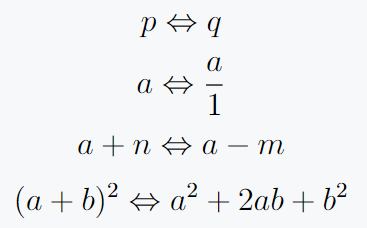This equation states that is equivalent to, indicating a bidirectional logical relationship between the two statements.

## Double Arrow (Implication)

The double arrow symbol can also be used to denote logical equivalence or a biconditional statement.

To insert this symbol, use the command \Longleftrightarrow within mathmode.

\documentclass{article}
\begin{document}
$(p \land q) \Longleftrightarrow (q \land p)$
$(a \land b) \Longleftrightarrow (b \land a)$
$a+b \Longleftrightarrow \frac{a+b}{n}$
$m\times n \Longleftrightarrow p\times q$
\end{document}

Output :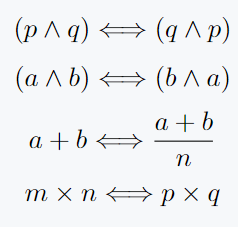This equation states that the conjunction of and is logically equivalent to the conjunction of and, representing a biconditional statement.

## Double Rightwards Arrow (Implies)

The double rightwards arrow symbol is used to indicate an implication or logical consequence.

To insert this symbol, use the command \Longrightarrow within mathmode.

\documentclass{article}
\begin{document}
$p \Longrightarrow q$
$x \Longrightarrow \int x\,dx$
$f(x) \Longrightarrow \sum_{i=1}^{n}\frac{x+i}{ni}$
\end{document}

Output :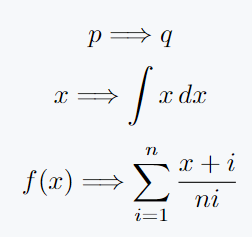This equation represents the logical implication that if is true, then must also be true.

## Double Leftwards Arrow (Implied by)

The double leftwards arrow symbol is used to indicate that a statement is implied or follows from another statement.

To insert this symbol, use the command \Longleftarrowwithin math mode.

\documentclass{article}
\begin{document}
$q \Longleftarrow p$
$\frac{a}{n} \Longleftarrow \frac{b}{n}$
$nq \Longleftarrow mp$
$\int f(x)\,dx \Longleftarrow x$
\end{document}

Output :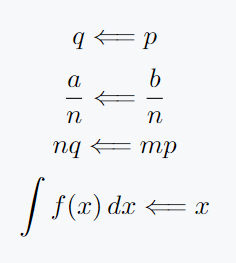This equation indicates that is implied by or follows from, representing a logical inference.

## Double Leftwards Arrow Over Rightwards Arrow (Equivalent to)

The double leftwards arrow over rightwards arrow symbol is used to represent equivalence or logical bi-implication.

\documentclass{article}
\usepackage{amssymb}
\begin{document}
$p \leftrightarrows q$
$\frac{a+b}{m} \leftrightarrows \frac{p+q}{n}$
$\int \frac{f(x)}{x} \, dx \leftrightarrows \sum_{x=1}^n \frac{f(x)}{x}$
\end{document}

Output :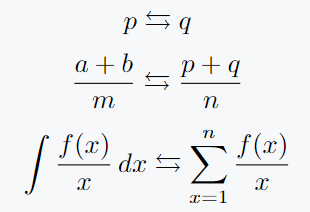## Double Rightwards Arrow Over Leftwards Arrow (Material implication)

The double rightwards arrow over leftwards arrow symbol is used to represent material implication or logical implication in one direction.

\documentclass{article}
\usepackage{amssymb}
\begin{document}
$p \rightleftarrows q$
$n \rightleftarrows \frac{n!}{(n-1)!}$
$\log \left(\frac{m}{n}\right) \rightleftarrows \log \left(\frac{p}{q}\right)$
\end{document} 

Output :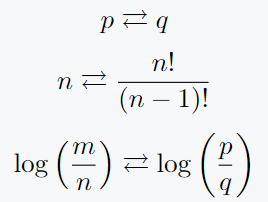## Conclusion

By employing the appropriate commands, you can indicate bidirectional relationships, logical equivalence, implications, and more within your mathematical equations or logical expressions.

Consider the specific context and intended meaning when choosing the most suitable double arrow symbol.

Enjoy incorporating double arrows into your LaTeX documents to express complex logical and mathematical relationships!#### Md Jidan Mondal

LaTeX expert with over 10 years of experience in document preparation and typesetting. Specializes in creating professional documents, reports, and presentations using LaTeX.